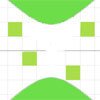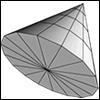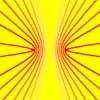# Resources tagged with: Conic Sections

There are 4 NRICH Mathematical resources connected to Conic Sections, you may find related items under Coordinates, Functions and Graphs.

Broad Topics > Coordinates, Functions and Graphs > Conic Sections### Lattice Points

##### Age 16 to 18 Challenge Level:

Why are there only a few lattice points on a hyperbola and infinitely many on a parabola?### Conic Sections

##### Age 16 to 18

The interplay between the two and three dimensional Euclidean geometry of conic sections is explored in this article. Suitable for students from 16+, teachers and parents.### Ellipses

##### Age 14 to 18 Challenge Level:

Here is a pattern for you to experiment with using graph drawing software. Find the equations of the graphs in the pattern.### Grid Points on Hyperbolas

##### Age 16 to 18 Challenge Level:

Find a condition which determines whether the hyperbola y^2 - x^2 = k contains any points with integer coordinates.